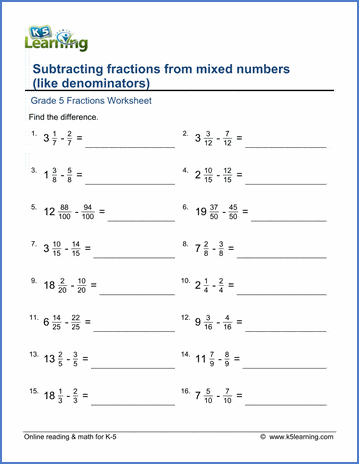# Subtracting fractions from mixed numbers

## Math worksheets: Subtracting fractions from mixed numbers with like denominators

Below are six versions of our grade 5 math worksheet on subtracting fractions from mixed numbers where the fractional part of the mixed number has the same denominator as the fraction being subtracted. These worksheets are pdf files.## More fractions worksheets

Explore all of our fractions worksheets, from dividing shapes into "equal parts" to multiplying and dividing improper fractions and mixed numbers.

## What is K5?

K5 Learning offers reading and math worksheets, workbooks and an online reading and math program for kids in kindergarten to grade 5.  We help your children build good study habits and excel in school.